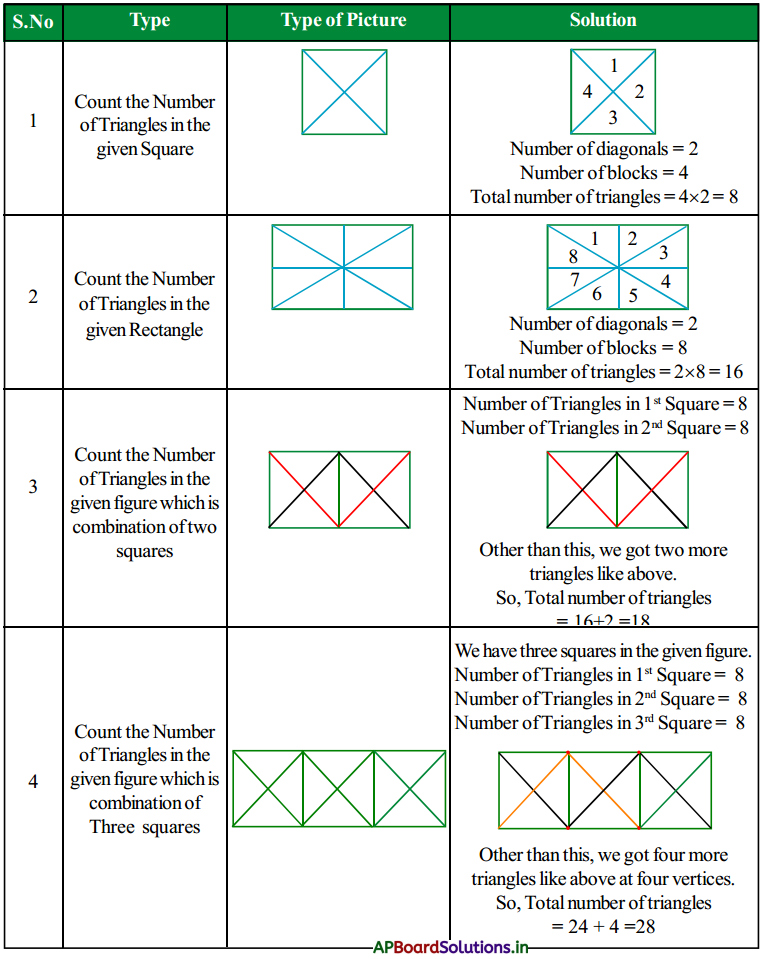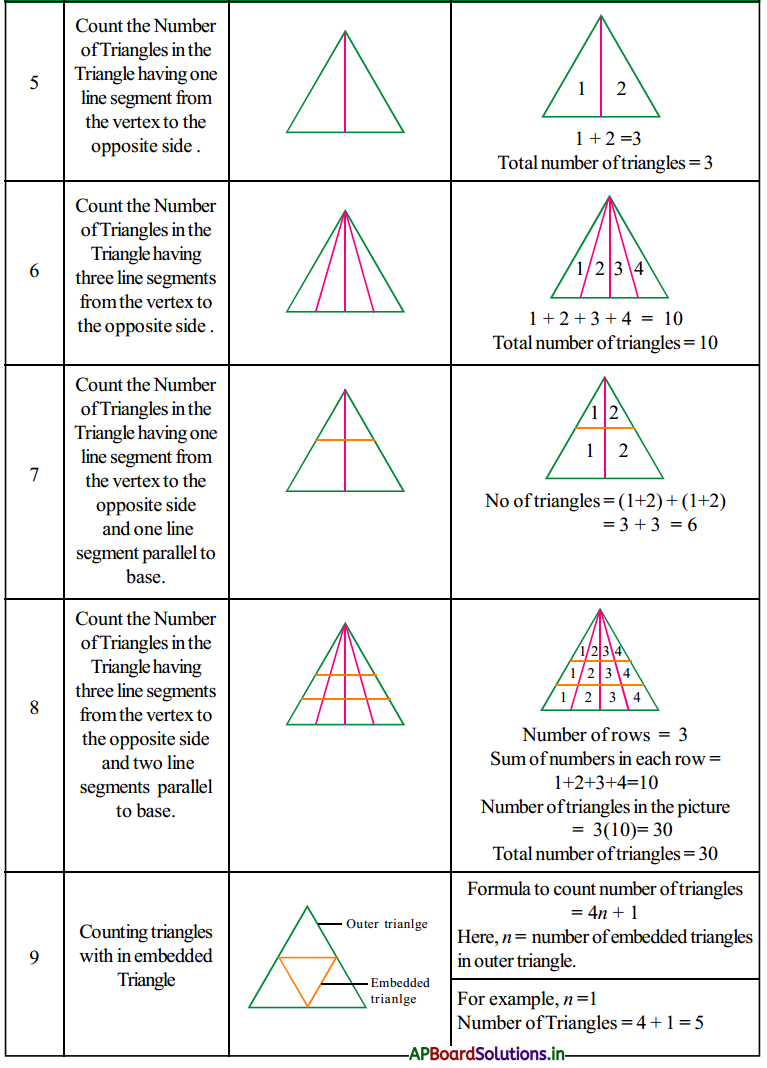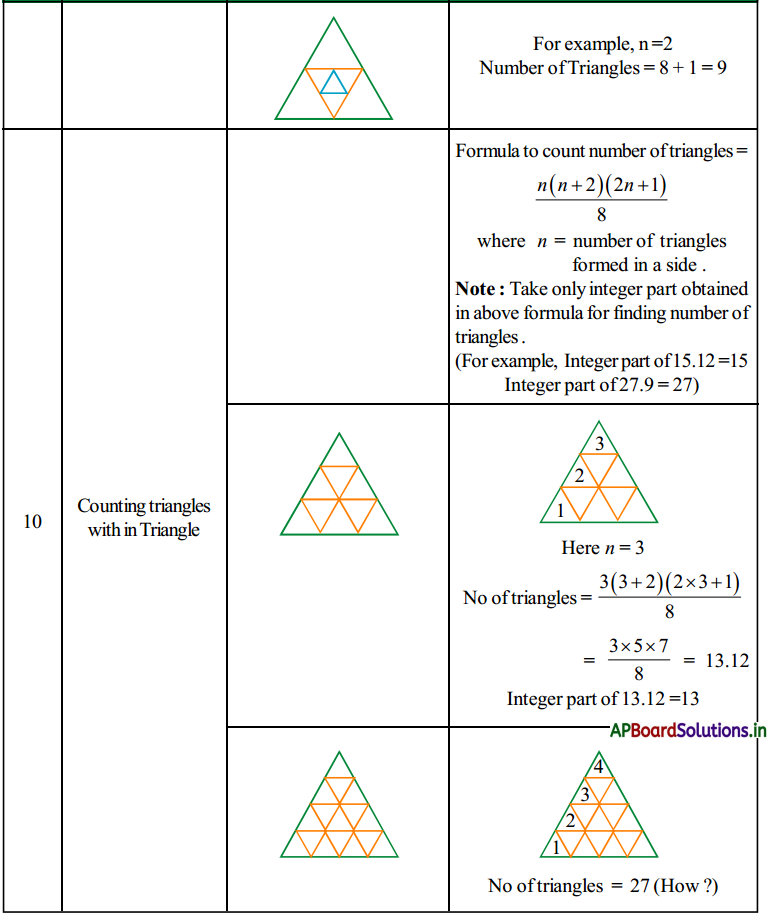# AP 7th Class Maths Notes 10th Lesson Construction of Triangles

Students can go through AP Board 7th Class Maths Notes 10th Lesson Construction of Triangles to understand and remember the concept easily.

## AP Board 7th Class Maths Notes 10th Lesson Construction of Triangles

→ The triangles can be constructed given the following cases :

• Three sides of a triangle.
• The lengths of any two sides and the measure of the angle between these sides.
• The measures of two angles and the lengths of side included between them.

→ To Construct a triangle we need at least the measure of one side.
Construct a triangle ABC given that AB = 4 cm, BC = 6 cm and AC = 5 cm.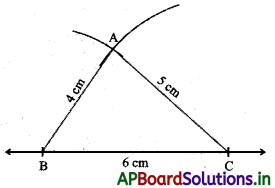Steps of construction:

• Draw a line segment BC = 6 cm.
• With B as centre and radius 4 cm, draw an arc.
• With C as centre and radius 5 cm, draw another arc, cutting the previous arc atA.
• Join BA and CA.
• ∆ABC is formed.→ Construction of a triangle given two sides and an included angle.
Construct triangle PQR given that PQ = 4cm,QR = 6.5cm and ∠Q = 60°.

Steps of construction: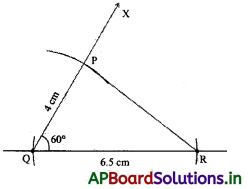• Draw a line segment QR = 6.5 cm.
• Using a protractor, draw ∠PQR = 60°.
• With Q as centre and radius 4 cm, draw an arc, cutting the ray $$\overrightarrow{\mathrm{QX}}$$ at P
• Join RP
• ∆PQR is formed.

→ Construction of a right angled triangle given a base and hypotenuse.
Construct a right angled triangle ABC given that AB = 4 cm, ∠A = 90°, AC = 3 cm.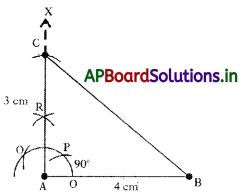For making angle 90:

• Take A as center and with any radius, draw an arc intersecting AB at O.
• Now O as center and same radius as before, draw an arc intersecting previous arc at P.
• Now P as center and same radius as before, draw an arc intersecting previous arc at Q.

Steps of Construction:

• Draw a line segment AB = 4 cm. .
• At A, draw an angle of 90° and name the line as AX;
• With A as centre and radius 3 cm, draw an arc cutting the line AX at C.
• Join BC.
• ∆ABC is formed.

→ Construction of a triangle given two angles and a side included between them.
Construct a triangle XYZ in which ∠X = 30°, ∠Y = 100° and XY = 6 cm.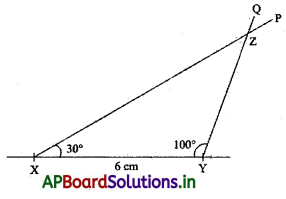Steps of Construction:

• Draw a line segment XY of length 6 cm.
• Using a protractor, draw ∠YXP = 30° at point X.
• Again using the protractor, draw ∠XYQ = 100° at point Q, intersecting the ray PX at Z.
• ∆XYZ is formed.→ Counting of Triangles